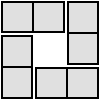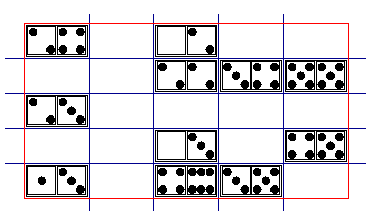#### You may also like### Domino Square

Use the 'double-3 down' dominoes to make a square so that each side has eight dots.### 4 Dom

Use these four dominoes to make a square that has the same number of dots on each side.### Eight Dominoes

Using the 8 dominoes make a square where each of the columns and rows adds up to 8

# Domino Magic Rectangle

### Domino Magic Rectangle

An ordinary set of 28 dominoes can be laid out as a 7 by 4 magic rectangle in which the spots in all the columns total 24 while the spots in all the rows total 42. Try it!

You may like to use this domino set or if you would prefer to use a computer interactivity, you might find our Dominoes Environment useful.

Printable NRICH Roadshow resources: Instructions and Gameboard

### Domino Magic Square

Magic squares can be made with the 25 dominoes remaining when you have put aside (0-5), (0-6) and (1-6). The total of each row, each column and each diagonal is 30. This can be done in many different ways. As a group project you might like to see how many distinct magic domino squares you can find.

Here is part of one layout to get you started.